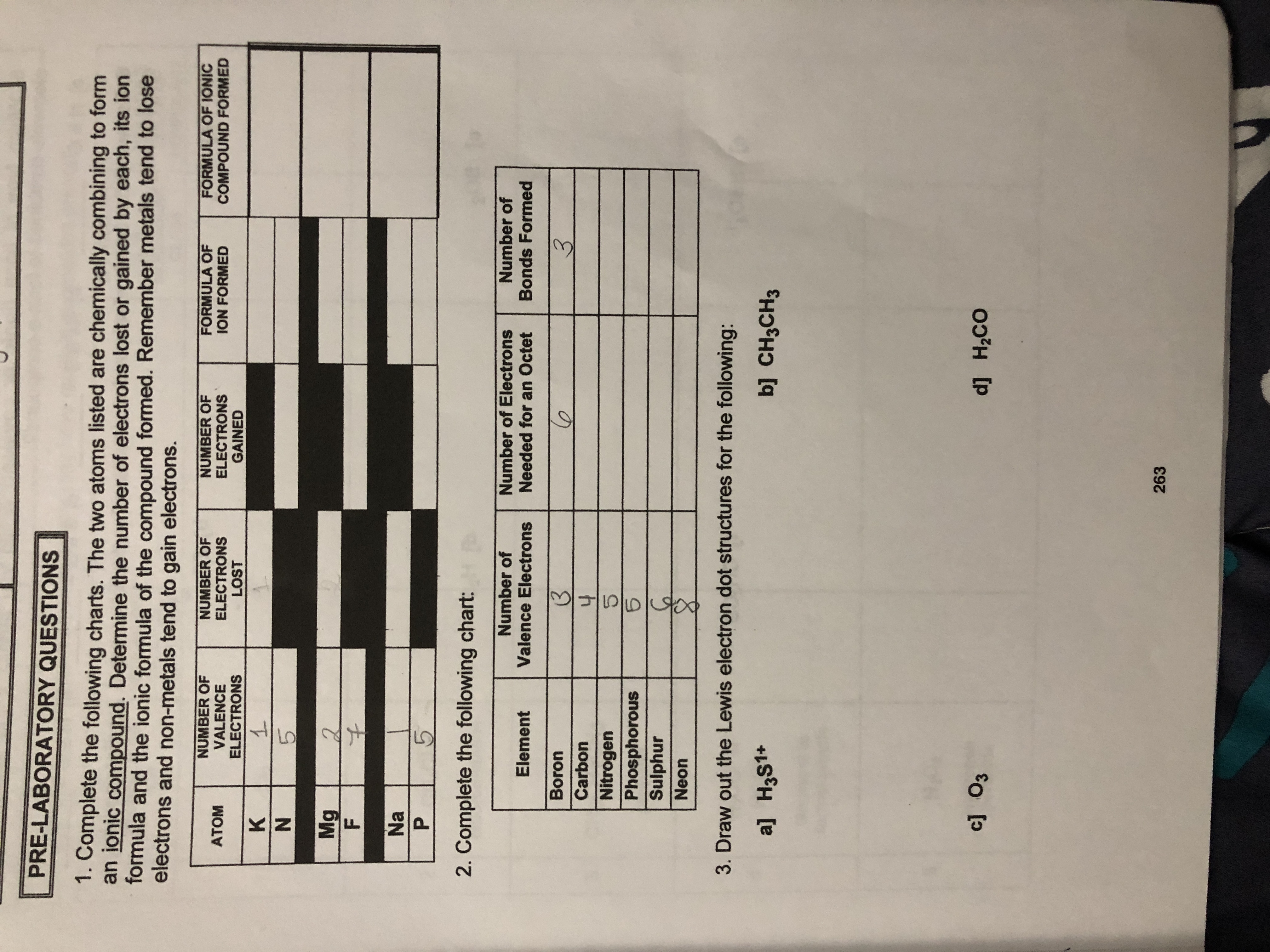# PRE-LABORATORY QUESTIONS1. Complete the following charts. The two atoms listed are chemically combining to formionic compound. Determine the number of electrons lost or gained by each, its ionformula and the ionic formula of the compound formed. Remember metals tend to loseelectrons and non-metals tend to gain electrons.NUMBER OFVALENCEELECTRONSNUMBER OFELECTRONSLOSTFORMULA OFION FORMEDNUMBER OFELECTRONSGAINEDFORMULA OF IONICCOMPOUND FORMEDАТОМ15MgF2NaP52. Complete the following chart:Number ofValence ElectronsNumber ofNumber of ElectronsNeeded for an OctetElementBonds FormedBoron3Carbon4NitrogenCIPhosphorousSulphurNeon3. Draw out the Lewis electron dot structures for the following:a] H3S1+b] CH3CH3c] O3d H2co263KN

Question
17 views

Question 1help_outlineImage TranscriptionclosePRE-LABORATORY QUESTIONS 1. Complete the following charts. The two atoms listed are chemically combining to form ionic compound. Determine the number of electrons lost or gained by each, its ion formula and the ionic formula of the compound formed. Remember metals tend to lose electrons and non-metals tend to gain electrons. NUMBER OF VALENCE ELECTRONS NUMBER OF ELECTRONS LOST FORMULA OF ION FORMED NUMBER OF ELECTRONS GAINED FORMULA OF IONIC COMPOUND FORMED АТОМ 1 5 Mg F 2 Na P 5 2. Complete the following chart: Number of Valence Electrons Number of Number of Electrons Needed for an Octet Element Bonds Formed Boron 3 Carbon 4 Nitrogen CI Phosphorous Sulphur Neon 3. Draw out the Lewis electron dot structures for the following: a] H3S1+ b] CH3CH3 c] O3 d H2co 263 KN fullscreen
check_circle

Step 1

The valence electrons of an atom are present in the outer most shell. An atom loses or gains electrons to make a stable electronic configuration. The sodium, potassium, and magnesium are metals. Sodium (Na) and potassium (K) belong to group 1 and they contain one valence electrons. Magnesium belongs to group 2 and it contains two valence electrons. Na and ...

### Want to see the full answer?

See Solution

#### Want to see this answer and more?

Solutions are written by subject experts who are available 24/7. Questions are typically answered within 1 hour.*

See Solution
*Response times may vary by subject and question.
Tagged in

### Chemistry